# 一类具有非线性脉冲的捕食与被捕食系统的定性分析*

(1.西安工业大学 理学院, 西安 710021; 2.陕西师范大学 数学与信息科学学院, 西安 710062)

## 引 言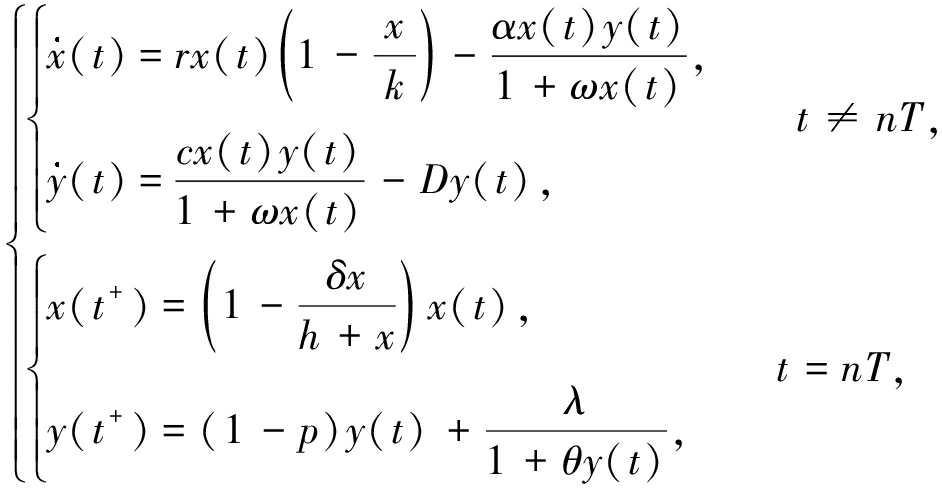(1)

## 1 预备知识和基本引理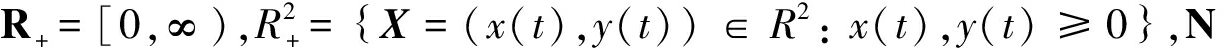表示非负整数集f=(f1, f2)为系统(1)前两个方程的右端函数,显然f的光滑性保证了解的存在唯一性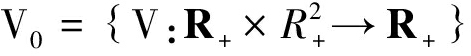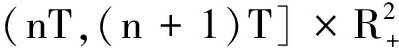上连续，nN,且lim(t,y)→(nT+,x)V(t,y)=V(nT+,x)存在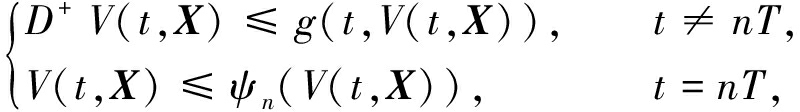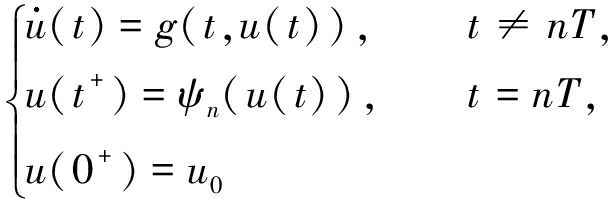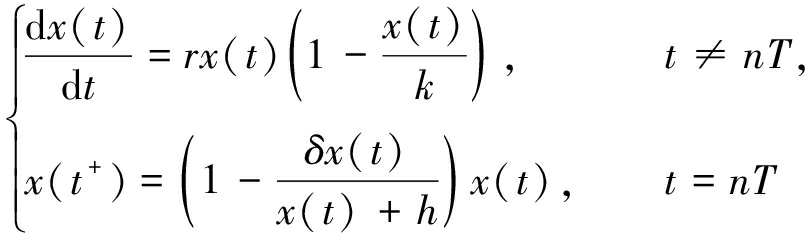(2)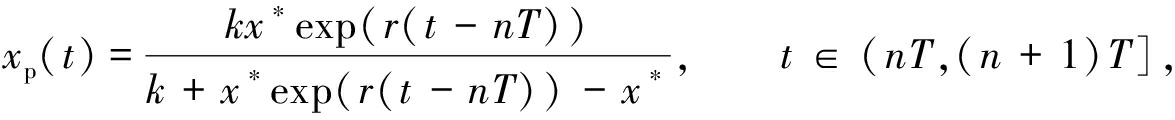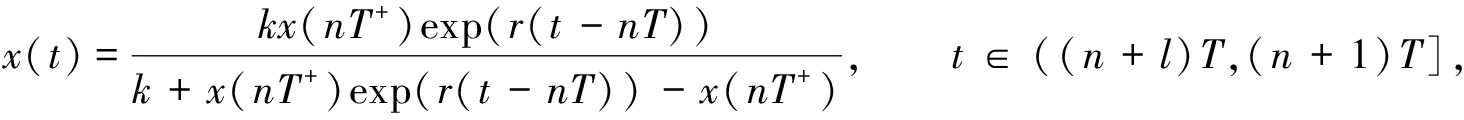x((n+1)T+)=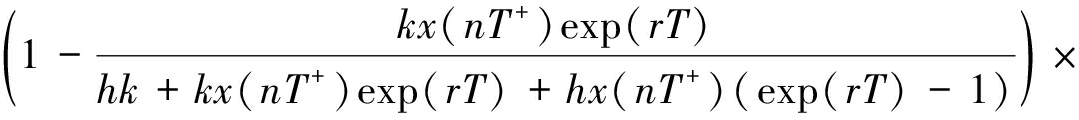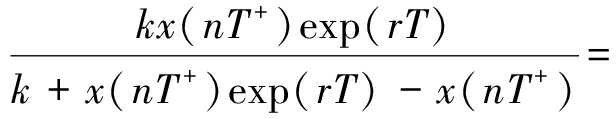F(x(nT+))

(3)

y((n+1)T+)=y(nT+),得到上面差分方程的唯一正稳点为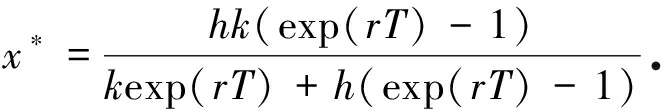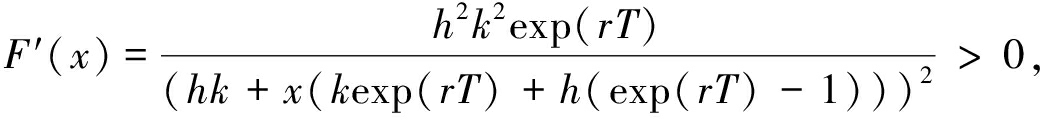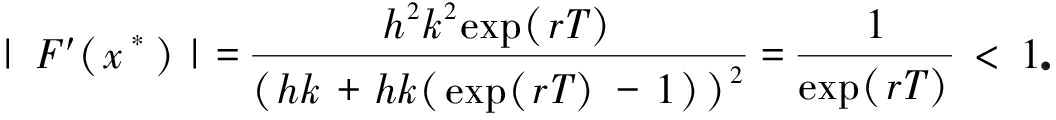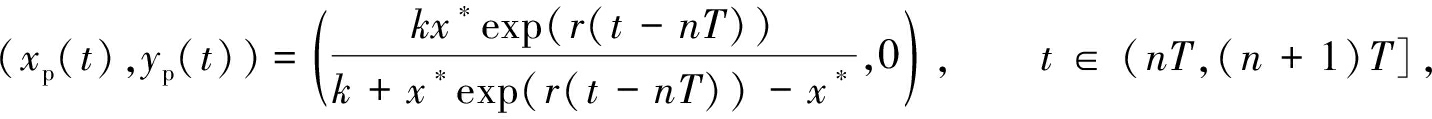## 2 天敌根除周期解的稳定性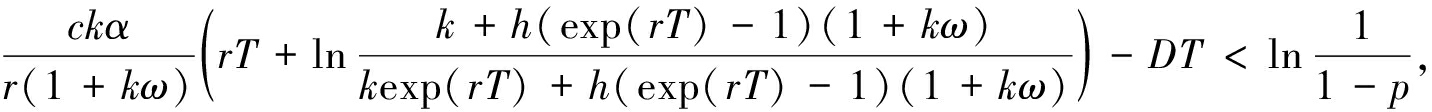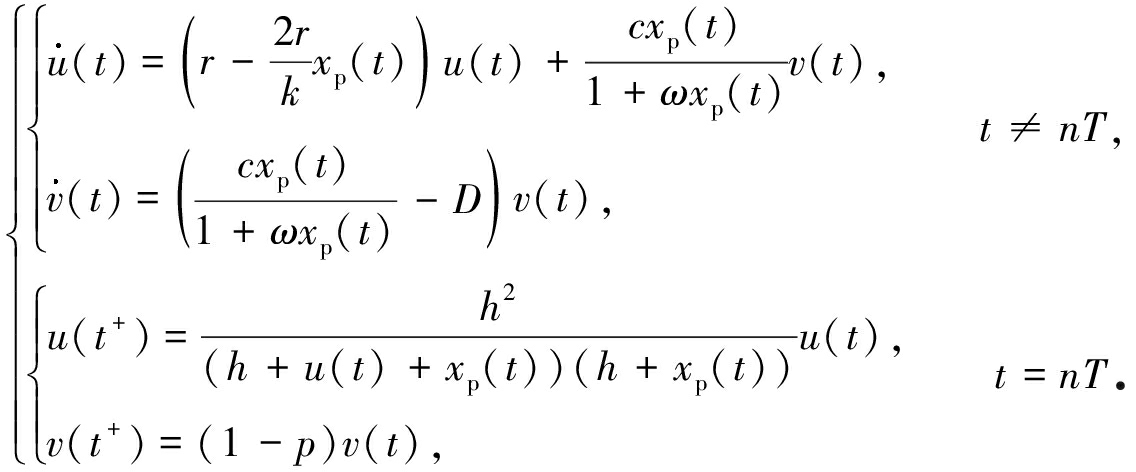(4)

φ(t)为系统(4)的基解矩阵,则φ(t)满足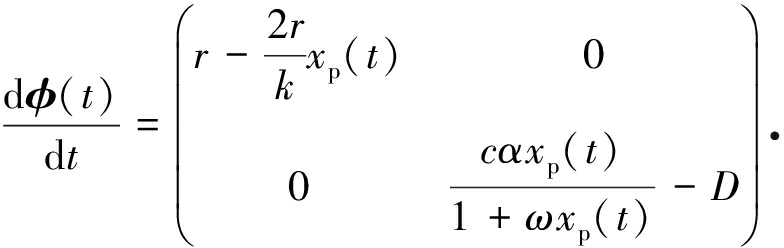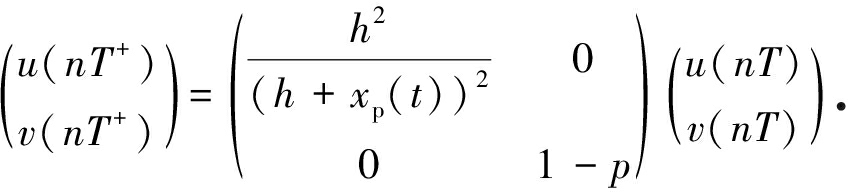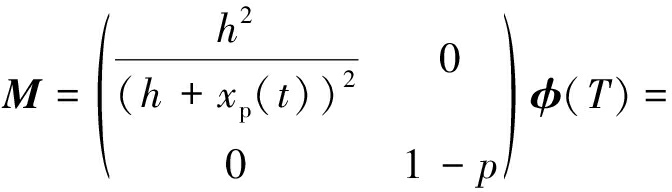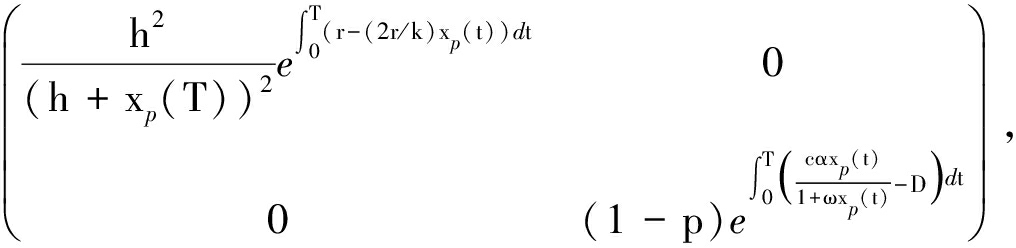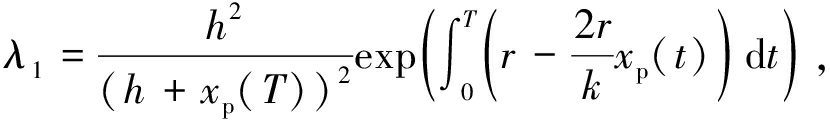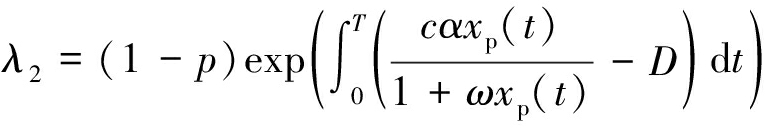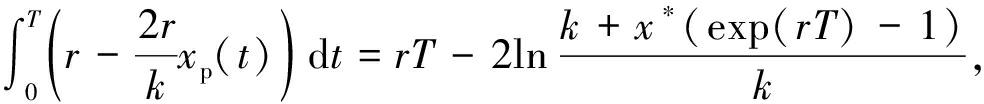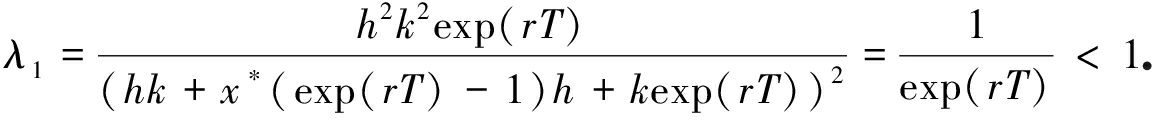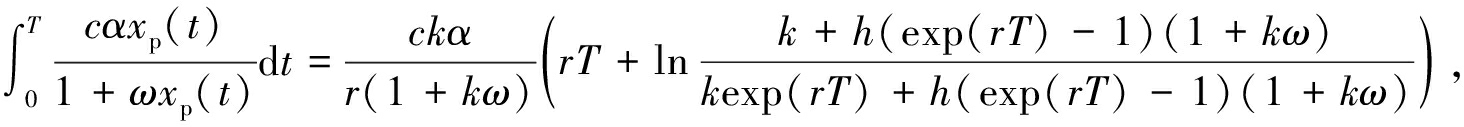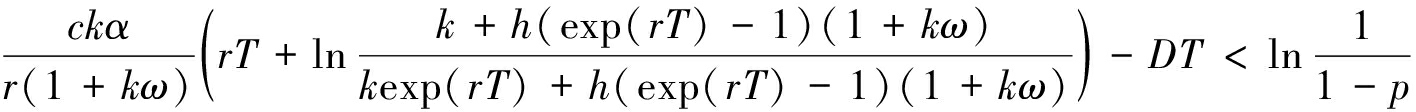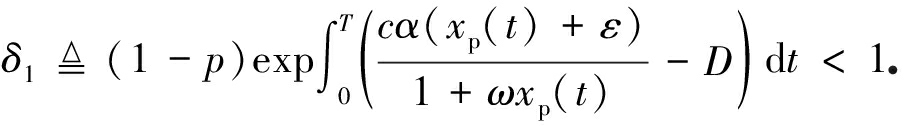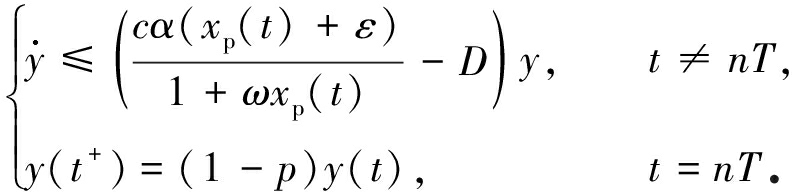(5)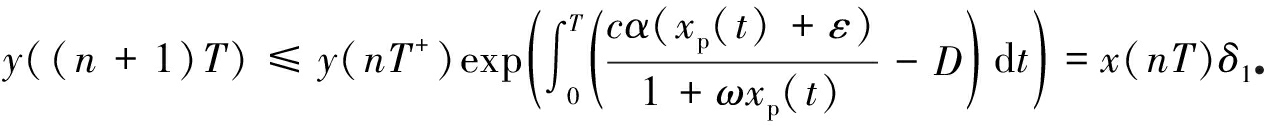(6)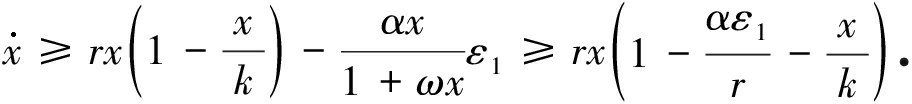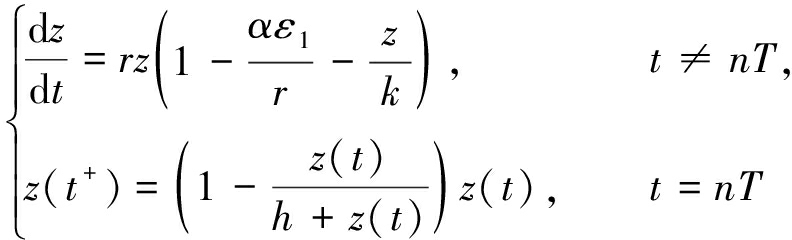(7)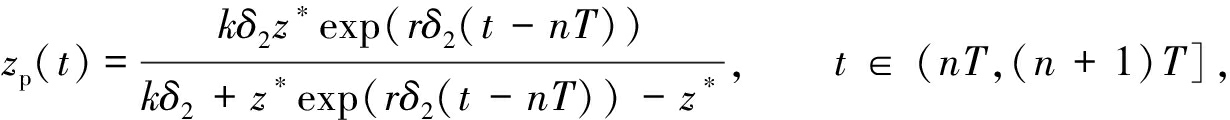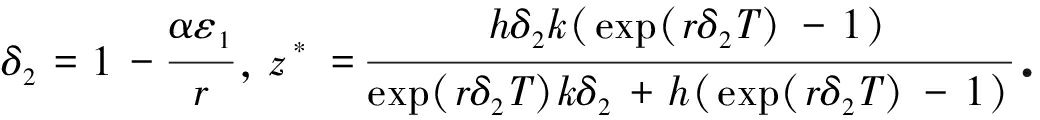## 3 非平凡周期解的存在性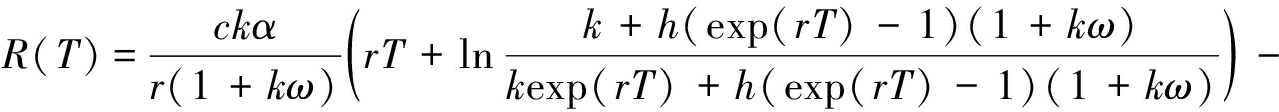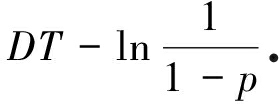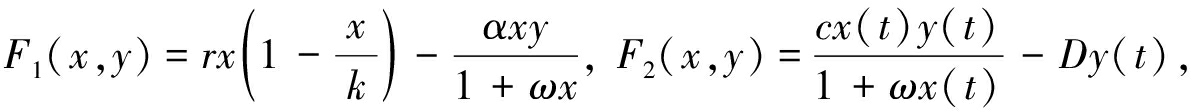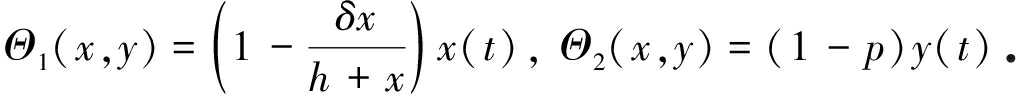Φ为上面方程有关的流,从而X(t)=Φ(t,X0)(0<tT),其中X0=(x(0),y(0)),X(T)=Φ(T,X0),ξ(t)=(xp(t),0)由文献有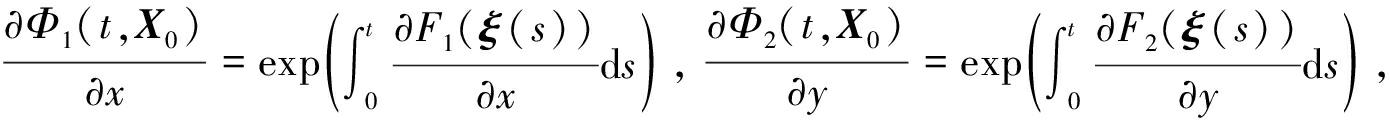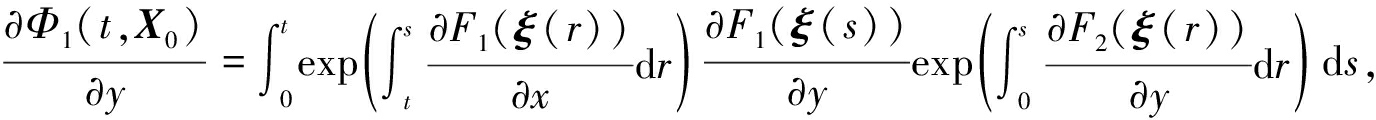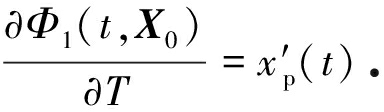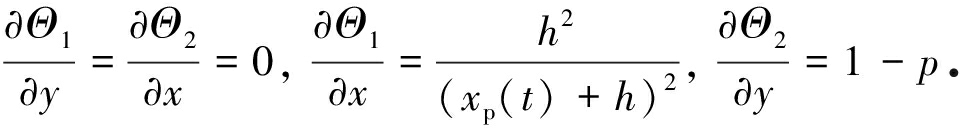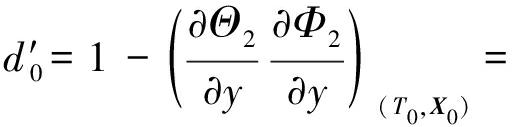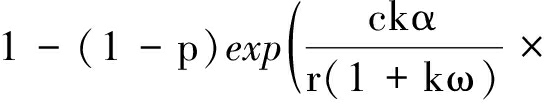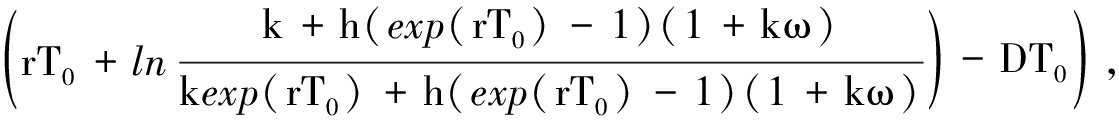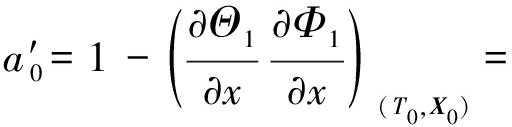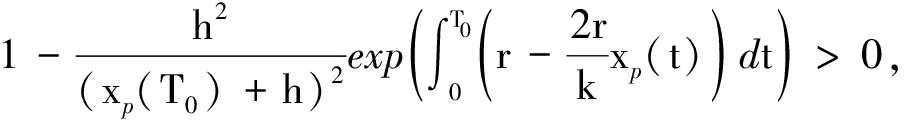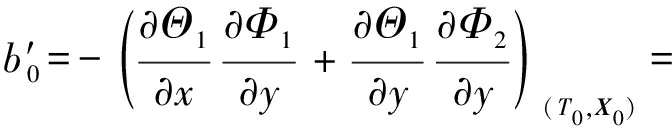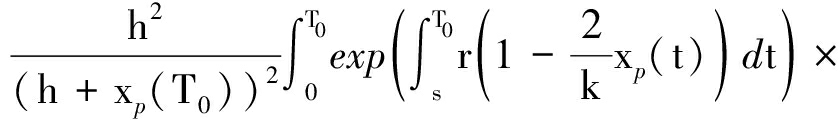c0=0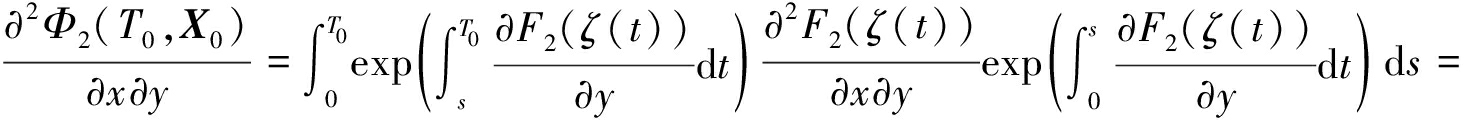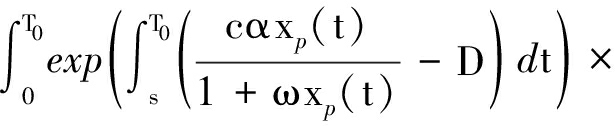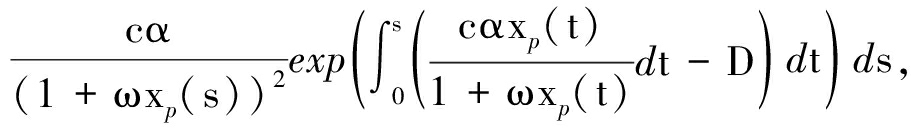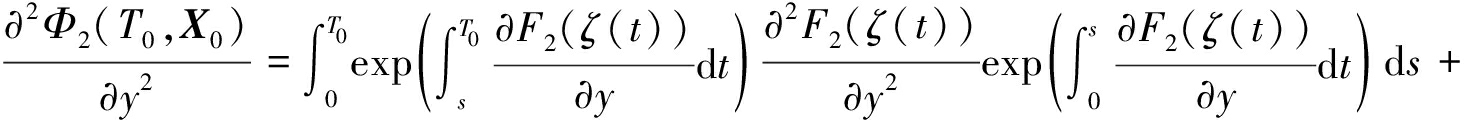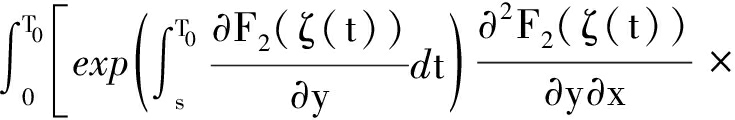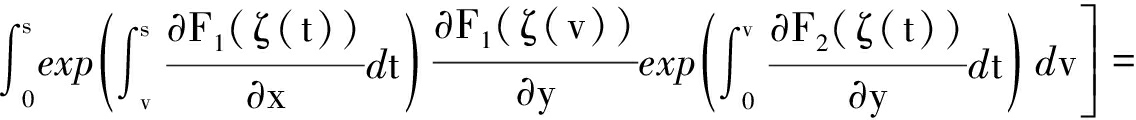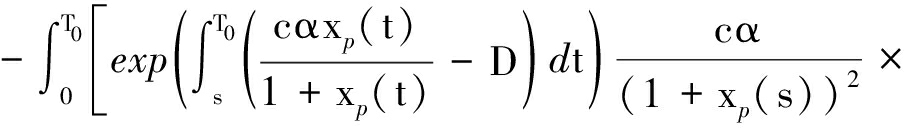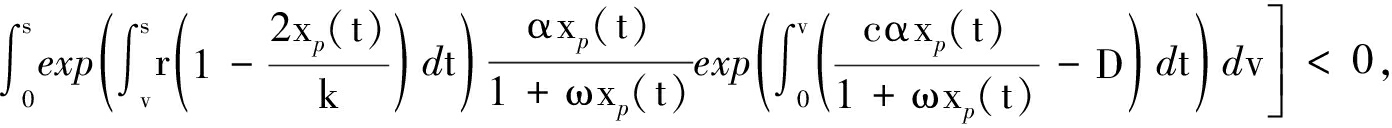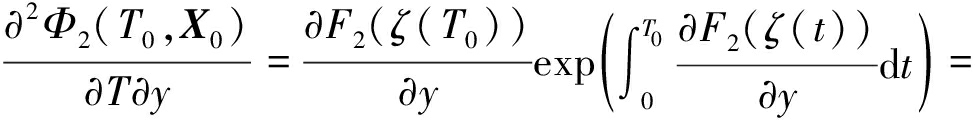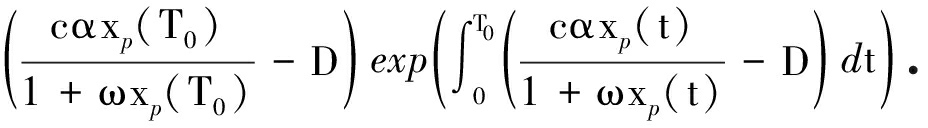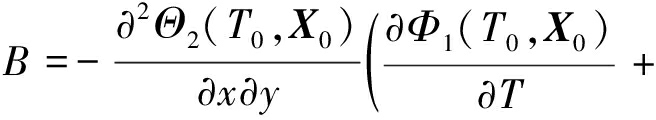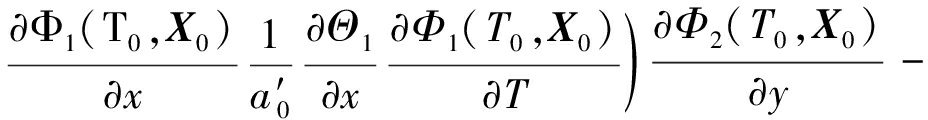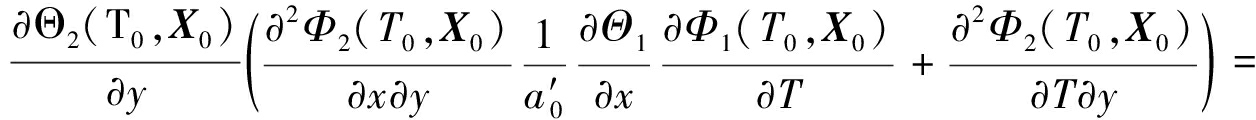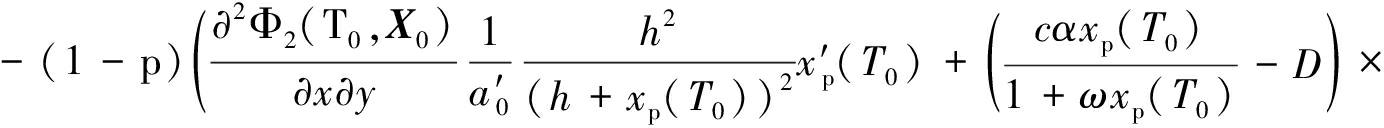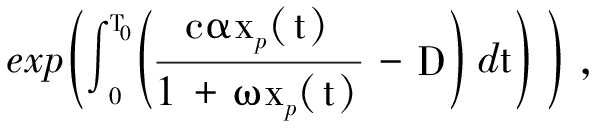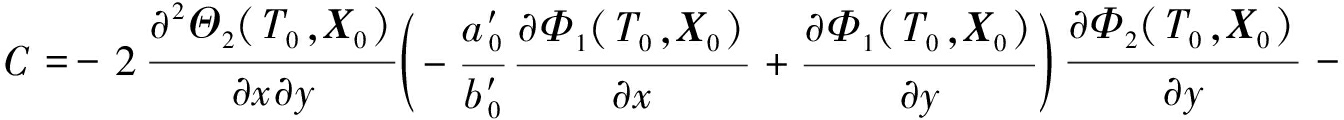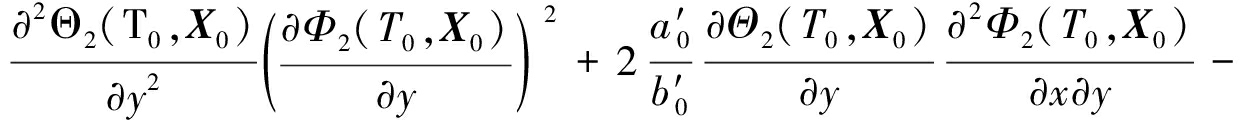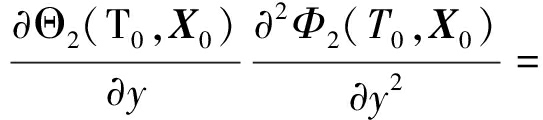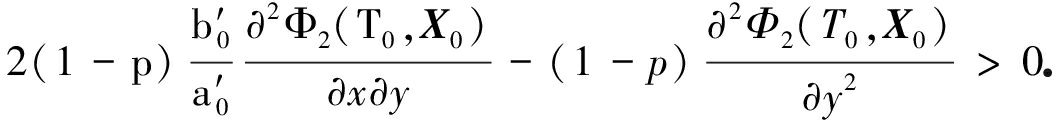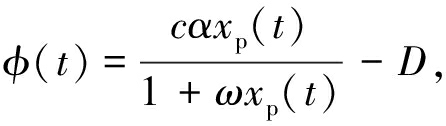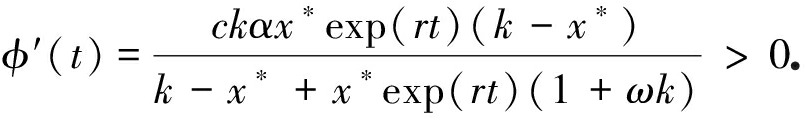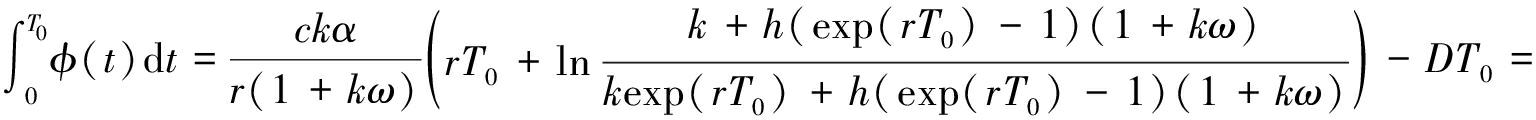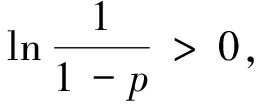## 4 数 值 模 拟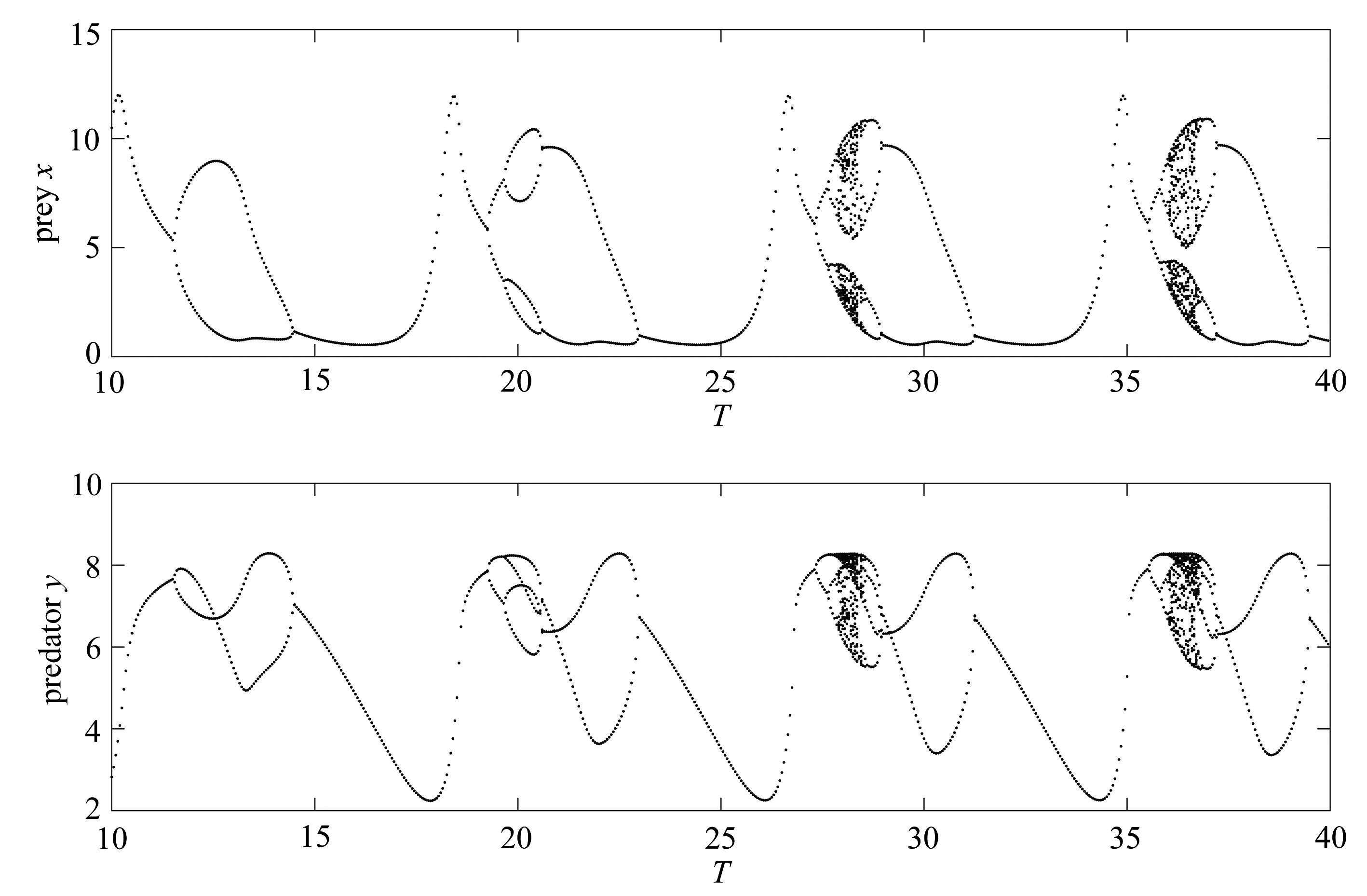Fig.1 Bifurcation diagrams of model (1) with respect to period T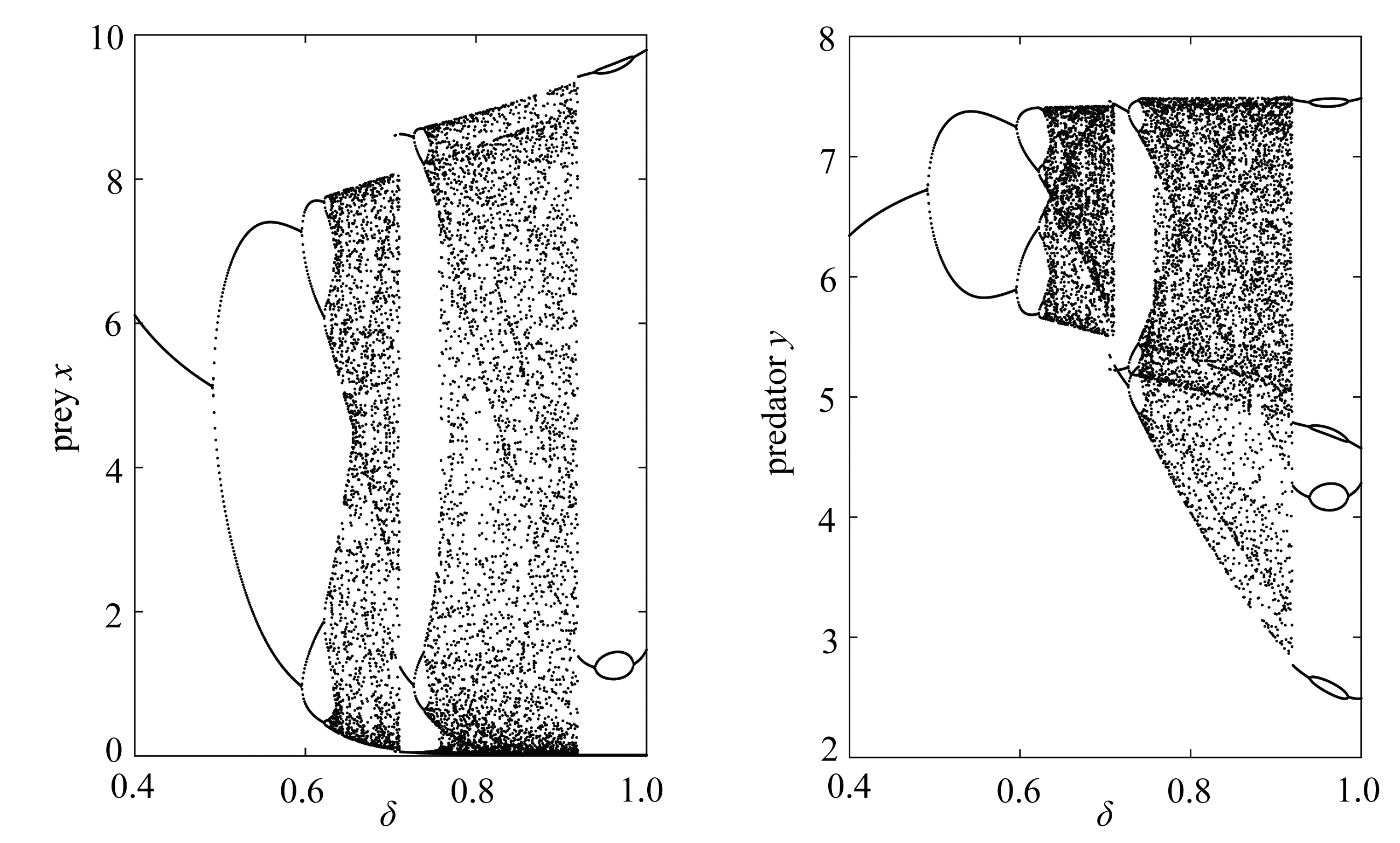Fig.2 Bifurcation diagrams of model (1) with respect to kill rate δ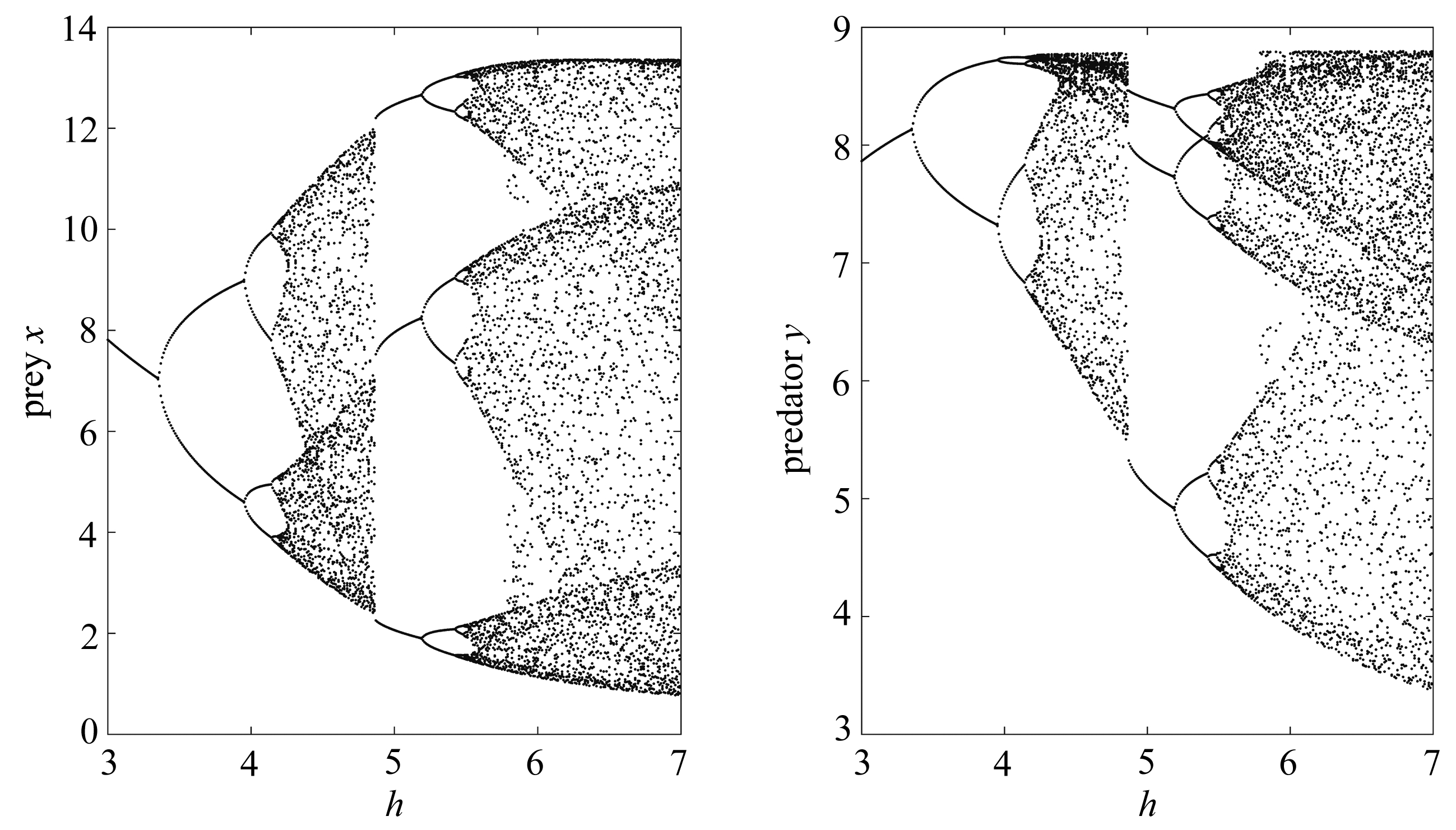Fig.3 Bifurcation diagrams of model (1) with respect to bifurcation parameter h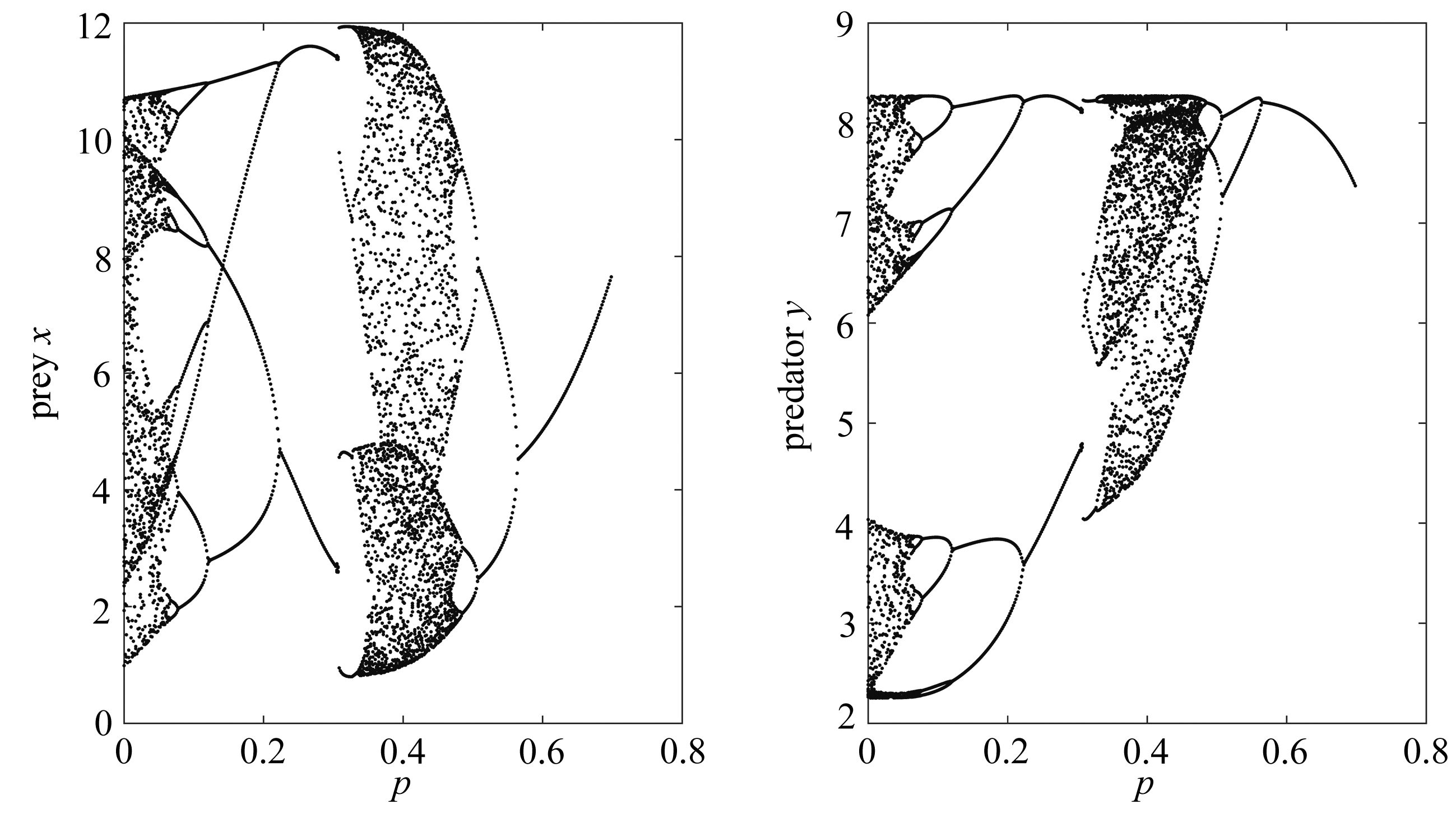Fig.4 Bifurcation diagrams of model (1) with respect to bifurcation parameter p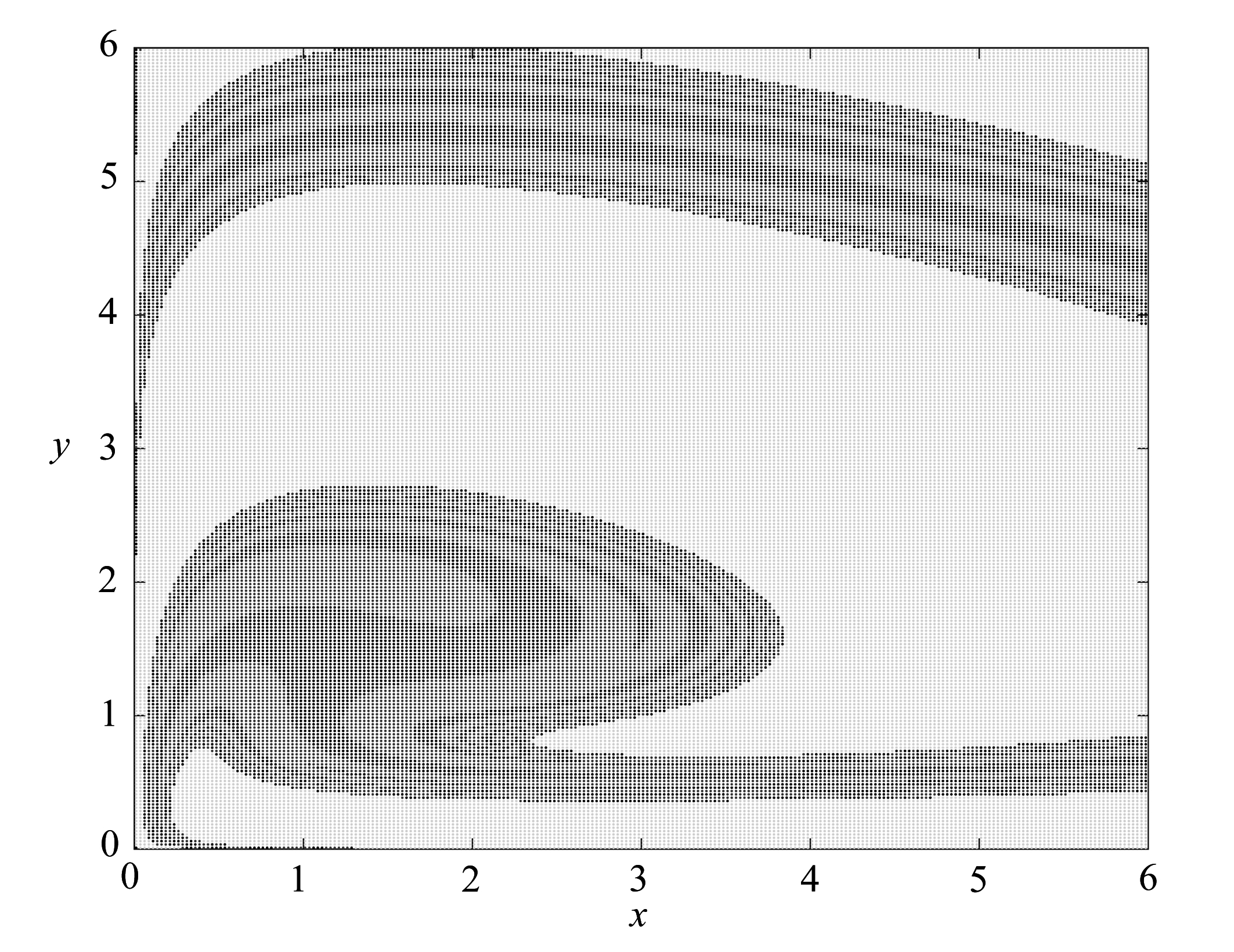Fig.5 The attraction basin of the three attractors of model (1)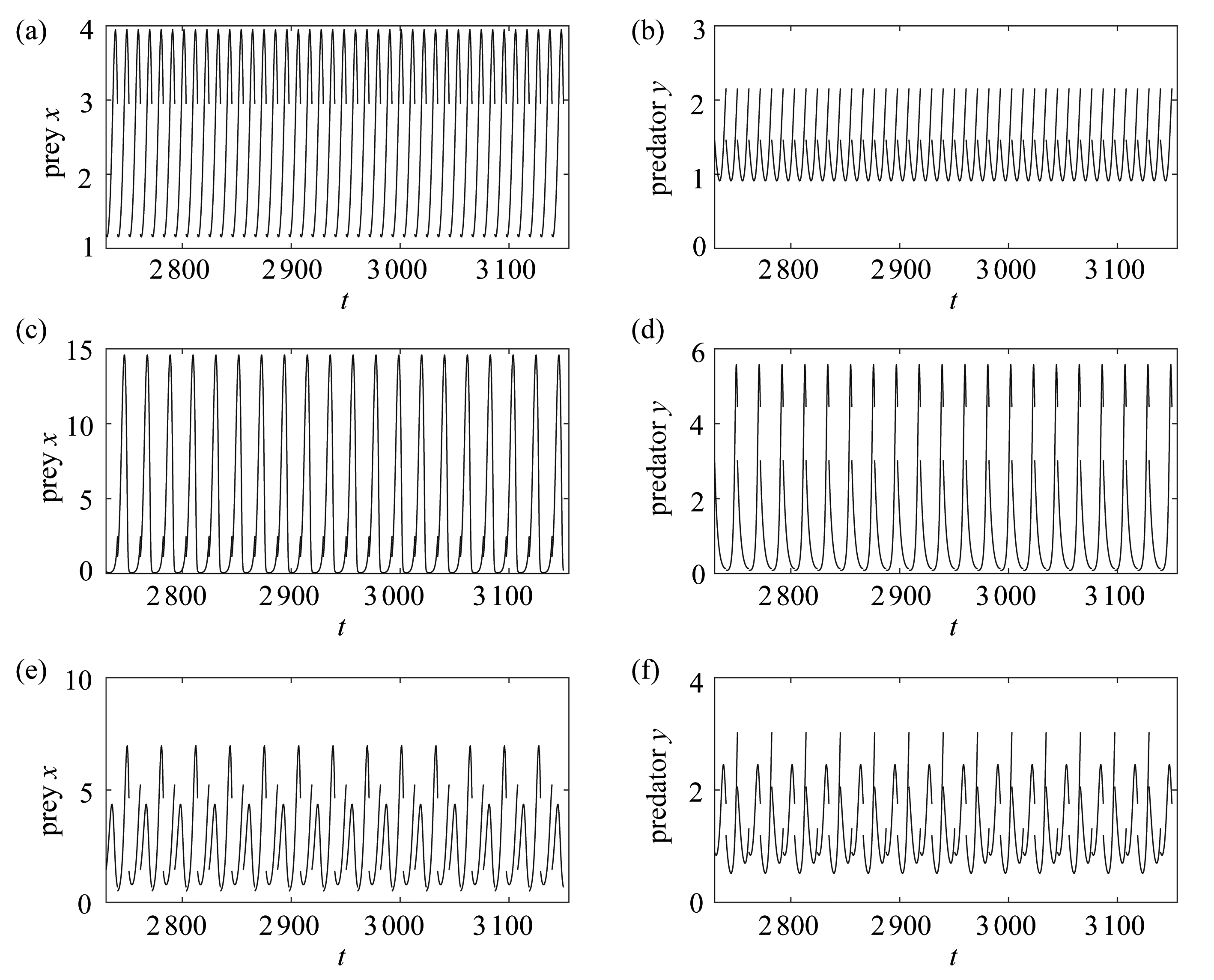Fig.6 Three coexistent stable attractors of model (1)

## 5 结 论

 VAN LENTEREN J C.Integrated pest management in protected crops[M]//Integrated Pest Management.DENT D, ed.London: Chapman & Hall, 1995.

 LIU B, ZHANG Y J, CHEN L S.The dynamical behaviors of a Lotka-Volterra predator-prey model concerning integrated pest management[J].Nonlinear Analysis Real World Applications, 2005, 6(2): 227-243.

 TANG S Y, CHEN L S.Density-dependent birth rate, birth pulses and their population dynamic consequences[J].Journal of Mathematical Biology, 2002, 44(2): 185-199.

 TANG S Y, CHEN L S.Multiple attractors in stage-structured population models with birth pulses[J].Bulletin of Mathematical Biology, 2003, 65(3): 479-495.

 TANG S Y, CHEN L S.The effect of seasonal harvesting on stage-structured populations models[J].Journal of Mathematical Biology, 2004, 48(4): 357-374.

 TANG S Y, XIAO Y N, CHEN L S, et al.Integrated pest management models and their dynamical behavior[J].Bulletin of Mathematical Biology, 2005, 67(1): 115-121.

 GAO W, TANG S Y.The effects of impulsive releasing methods of natural enemies on pest control and dynamical complexity[J].Nonlinear Analysis: Hybrid Systems, 2011, 5(3): 540-553.

 LI C T, TANG S Y.The effects of timing of pulse spraying and releasing periods on dynamics of generalized predator-prey model[J].International Journal of Biomathematics, 2012, 5(1): 1-27.

 TANG S Y, LIANG J H.Global qualitative analysis of a non-smooth Gause predator-prey model with a refuge[J].Nonlinear Analysis, 2013, 76(1): 165-180.

 BAEK H K.Qualitative analysis of Beddington-DeAngelis type impulsive predator-prey models[J].Nonlinear Analysis: Real World Applications, 2010, 11: 1312-1322.

 王小娥, 蔺小林, 李建全.一类具有脉冲免疫治疗的HIV-1感染模型的动力学分析[J].应用数学和力学, 2019, 40(7): 728-740.(WANG Xiaoe, LIN Xiaolin, LI Jianquan.Dynamic analysis of a class of HIV-1 infection models with pulsed immunotherapy[J].Applied Mathematics and Mechanics, 2019, 40(7): 728-740.(in Chinese))

 王刚, 唐三一.非线性脉冲状态依赖捕食被捕食模型的定性分析[J].应用数学和力学, 2013, 34(5): 496-505.(WANG Gang, TANG Sanyi.Qualitative analysis of prey-predator model with nonlinear impulsive effects[J].Applied Mathematics and Mechanics, 2013, 34(5): 496-505.(in Chinese))

 YANG J, TANG G Y, TANG S Y.Holling type Ⅱ predator-prey model with nonlinear pulse as state-dependent feedback control[J].Journal of Computational and Applied Mathematics, 2016, 291(1): 225-241.

 TIAN Y, TANG S Y, CHEKE R A.Dynamic complexity of a predator-prey model for IPM with nonlinear impulsive control incorporating a regulatory factor for predator releases[J].Mathematical Modelling and Analysis, 2019, 24(1): 134-154.

 LI C T, TANG S Y.Analyzing a generalized pest-natural enemy model with nonlinear impulsive control[J].Open Mathematics, 2018, 16(1): 1390-1411.

 LAKMECHE A, ARINO O.Bifurcation of non-trivial periodic solutions of impulsive differential equations arising chemotherapeutic treatment[J].Dynamics of Continuous, Discrete and Impulsive Systems(Series A): Mathematical Analysis, 2000, 7(2): 265-287.

# Analysis of the Predator-Prey Model With Nonlinear Impulsive Control

LI Changtong1,2

(1.School of Science, Xian Technological University, Xian 710021, P.R.China； 2.School of Mathematics and Information Science, Shaanxi Normal University,Xian 710062, P.R.China)

Abstract: Due to limited resources and population densities, the actual pest control strategies such as spraying pesticide and releasing natural enemies have saturation effects or nonlinearity.Therefore, a predator-prey model with nonlinear impulsive control due to resource limitation was proposed and analyzed.With the Floquet theory and the differential comparison principle, the condition for global stability of the predator-free periodic solution was provided.It is shown that once a threshold condition is met, a stable nontrivial periodic solution will emerge via a supercritical bifurcation.The numerical results show that the predator-prey model with nonlinear pulse has rich dynamical behaviors.

Key words: globally stable; periodic solution; nonlinear pulse; pest control

*收稿日期： 2019-07-23；

ⓒ 应用数学和力学编委会，ISSN 1000-0887

http://www.applmathmech.cn

DOI: 10.21656/1000-0887.400226

Foundation item: The National Natural Science Foundation of China(61772017)